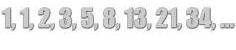# Exponents Lesson

## Learn about the rules of exponents with the following examples and interactive exercises.In the table below, the number 2 is written as a factor repeatedly. The product of factors is also displayed in this table. Suppose that your teacher asked you to Write 2 as a factor one million times for homework. How long do you think that would take? Answer.

 Factors Product of Factors Description 2 x 2 = 4 2 is a factor 2 times 2 x 2 x 2 = 8 2 is a factor 3 times 2 x 2 x 2 x 2 = 16 2 is a factor 4 times 2 x 2 x 2 x 2 x 2 = 32 2 is a factor 5 times 2 x 2 x 2 x 2 x 2 x 2 = 64 2 is a factor 6 times 2 x 2 x 2 x 2 x 2 x 2 x 2 = 128 2 is a factor 7 times 2 x 2 x 2 x 2 x 2 x 2 x 2 x 2 = 256 2 is a factor 8 times

Writing 2 as a factor one million times would be a very time-consuming and tedious task. A better way to approach this is to use exponents. Exponential notation is an easier way to write a number as a product of many factors.

BaseExponent

The exponent tells us how many times the base is used as a factor.

For example, to write 2 as a factor one million times, the base is 2, and the exponent is 1,000,000. We write this number in exponential form as follows:

21,000,000

read as two raised to the millionth powerExample 1: Write 2 x 2 x 2 x 2 x 2 using exponents, then read your answer aloud.

Solution: 2 x 2 x 2 x 2 x 2  =  25             2 raised to the fifth power

Let us take another look at the table from above to see how exponents work.

 Exponential Form Factor Form Standard Form 22 = 2 x 2 = 4 23 = 2 x 2 x 2 = 8 24 = 2 x 2 x 2 x 2 = 16 25 = 2 x 2 x 2 x 2 x 2 = 32 26 = 2 x 2 x 2 x 2 x 2 x 2 = 64 27 = 2 x 2 x 2 x 2 x 2 x 2 x 2 = 128 28 = 2 x 2 x 2 x 2 x 2 x 2 x 2 x 2 = 256

So far we have only examined numbers with a base of 2. Let's look at some examples of writing exponents where the base is a number other than 2.

Example 2: Write 3 x 3 x 3 x 3 using exponents, then read your answer aloud.

Solution: 3 x 3 x 3 x 3  =  34           3 raised to the fourth power

Example 3: Write 6 x 6 x 6 x 6 x 6 using exponents, then read your answer aloud.

Solution: 6 x 6 x 6 x 6 x 6  =  65           6 raised to the fifth power

Example 4: Write 8 x 8 x 8 x 8 x 8 x 8 x 8 using exponents, then read your answer aloud.

Solution: 8 x 8 x 8 x 8 x 8 x 8 x 8  =  87           8 raised to the seventh power

Example 5: Write 103, 36, and 18 in factor form and in standard form.

Solution:

 Exponential Form Factor Form Standard Form 103 10 x 10 x 10 1,000 36 3 x 3 x 3 x 3 x 3 x 3 729 18 1 x 1 x 1 x 1 x 1 x 1 x 1 x 1 1

The following rules apply to numbers with exponents of  0, 1, 2 and 3:

 Rule Example Any number (except 0) raised to the zero power is equal to 1. 1490 = 1 Any number raised to the first power is always equal to itself. 81 = 8 If a number is raised to the second power, we say it is squared. 32 is read as three squared If a number is raised to the third power, we say it is cubed. 43 is read as four cubed

Summary: Whole numbers can be expressed in standard form, in factor form and in exponential form. Exponential notation makes it easier to write a number as a factor repeatedly. A number written in exponential form is a base raised to an exponent. The exponent tells us how many times the base is used as a factor.

### Exercises

Directions: Read each question below. Click once in an ANSWER BOX and type in your answer; then click ENTER. Do not use commas in your answers, just digits. After you click ENTER, a message will appear in the RESULTS BOX to indicate whether your answer is correct or incorrect. To start over, click CLEAR.

 1 Write 45 in standard form. ANSWER BOX:   RESULTS BOX:
 2 Write 54 in standard form. ANSWER BOX:   RESULTS BOX:
 3 What is 500,000,000 raised to the zero power? ANSWER BOX:   RESULTS BOX:
 4 What is 237 raised to the first power? ANSWER BOX:   RESULTS BOX:
 5 The number 81 is 3 raised to which power? ANSWER BOX:   RESULTS BOX: